Courses

WBJEE Maths Test - 1

75 Questions MCQ Test WBJEE Sample Papers, Section Wise & Full Mock Tests | WBJEE Maths Test - 1

Description
Attempt WBJEE Maths Test - 1 | 75 questions in 120 minutes | Mock test for JEE preparation | Free important questions MCQ to study WBJEE Sample Papers, Section Wise & Full Mock Tests for JEE Exam | Download free PDF with solutions
QUESTION: 1

Solution:
QUESTION: 2

Solution:
QUESTION: 3

The third term in the expansion of ((x2) - (1/x3))n is independent of x, when n is equal to

Solution:
QUESTION: 4

The equation of the circle passing through intersection of circles x2+y2-1=0 and x2+y2-2x-4y+1=0 and touching x+2y=0 is

Solution:
QUESTION: 5

The square root of the number 5 + 12i is

Solution:
QUESTION: 6

The equation of circle which passes through (4,5) and whose centre is (2,2) is

Solution:
QUESTION: 7

The volume of a solid which is obtained by revolving area bounded by an ellipse x2+9y2=9 and straight line x+3y=3 about y-axis is

Solution:
QUESTION: 8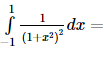Solution:
QUESTION: 9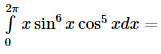Solution:
QUESTION: 10

If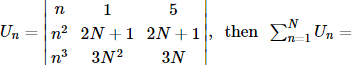Solution:
QUESTION: 11

The degree of the differential equation d2y/dx2+(dy/dx)3+6y=0 is

Solution:
QUESTION: 12

The roots of the equation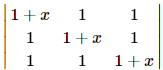=0 are

Solution: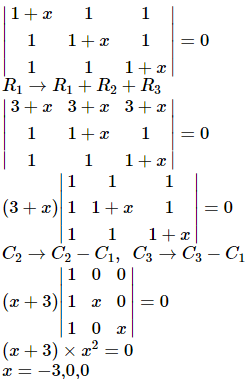QUESTION: 13

Differential of x6 w.r. to x3 is equal to

Solution:
QUESTION: 14
The solution of the differential equation 2x dy/dx-y=3 represent
Solution:
QUESTION: 15
If |z| = 4 and arg z = (5π/6), then the value of z is
Solution:
QUESTION: 16

If x = a cos3 θ, y = a sin3 θ, then
√[1 + (dy/dx)2] =

Solution:
QUESTION: 17

The eccentricity of the conic 9x2 + 25y2 = 225 is

Solution:
QUESTION: 18

The graph represented by the equations x = sin2t,y = 2cost is

Solution:
QUESTION: 19

If the major axis of an ellipse is thrice the minor axis, then its eccentricity is equal to

Solution:
QUESTION: 20

The curve represented by x = a (coshθ + sinhθ) , y = b(coshθ − sinhθ) is

Solution:
QUESTION: 21

The least possible value of k for which the function f(x) = x2 + kx + 1 may be increasing on [1,2] is

Solution:

We have , f x = x 2 + k x + 1
⇒ f ′ x = 2 x + k . Also , f ″ x = 2
Now , f ″ x = 2, ∀ x ∈ [ 1,2 ]
⇒ f ″ x > 0, ∀ x ∈ [ 1,2 ]
⇒ f ′ x is an increasing function in the interval [ 1,2 ] .
⇒ f ′ 1 is the least value of f′(x) on [ 1,2 ]
But f ′ x > 0 ∀ x ∈ [ 1,2 ]
[ ∵ f x is increasing on [1,2] ]
∴ f ′ 1 > 0, ∀ x ∈ [ 1,2 ] ⇒ k > − 2
Thus, the least value of k is -2

QUESTION: 22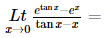Solution:
QUESTION: 23
The system of equations
αx+y+z = α-1
x+αy+z = α-1
x+y+αz, = α-1 has no solution, if α is
Solution:
QUESTION: 24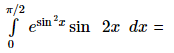Solution: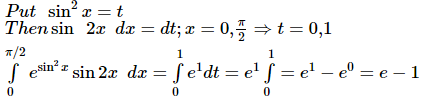QUESTION: 25

If z=1+i,then the multiplicative inverse of z2 is

Solution:
QUESTION: 26
If a, b, c and d are four positive real numbers such that abcd = 1, what is the minimum value of (1 + a)(1 + b)(1 + c)(1 + d)?
Solution:
QUESTION: 27

The equation of the parabola whose vertex is at (2,-1) and focus at (2,-3) is

Solution:
QUESTION: 28

sin[(1/2)cos⁻1(4/5)] is equal to

Solution:
QUESTION: 29

The pole of the line 2x + 3y − 4 = 0 with respect to the parabola y2 = 4x is

Solution:
QUESTION: 30

How many total words can be formed from the letters of the word INSURANCE in which vowels are always together?

Solution:
QUESTION: 31
Number of triangles formed by joining 12 points, no three of which are in the same straight line except 7 of them which are in a straight line, is
Solution:
QUESTION: 32

The probability of drawing a card which is atleast a spade or a king from a well shuffled pack of cards is

Solution:

Let A be the event that the card drawn is spade,
B be the event that card drawn is king and S be the sample space,
When a card is drawn from a pack of cards.
Now n(S) = 52C1 = 52, n(A) = 13C1 = 13, n(B) = 4C1 = 4, n(A ∩ B) = 1C1 = 1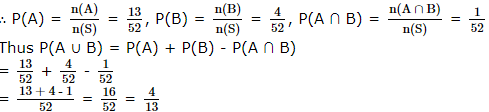QUESTION: 33
A speak the truth in 75% cases and B in 80% cases. In what percent of cases are they likely to contradict each other narring the same incident is
Solution:
QUESTION: 34
If 56Pr+6 : 54Pr+3=30800 : 1, then
Solution:
∵ 56Pr + 6 : 54Pr + 3 = 30800 : 1
⇒ 56! / (50 - r)! = (30800 * 54!) / (51-r!)
⇒ 56 x 55 = 30800/(51 - r)
⇒ 51 - r = 10
∴ r = 41
QUESTION: 35

In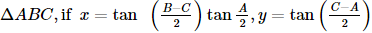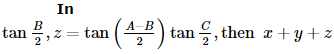(in terms of x , y , z only)  is

Solution:
QUESTION: 36

In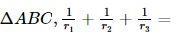Solution:
QUESTION: 37
What is the probability of getting a sum 9 from two throws of dice?
Solution:
QUESTION: 38
If α and β are the roots of x2 + px + 1 = 0 and γ and δ are the roots of x2 + qx + 1 = 0, the value of (α - γ)(β - γ)(α + δ)(β + δ)
Solution: x2 + px + 1 = 0 has roots α, β
x2 + qx + 1 = 0 has roots γ, δ
Using the sum and product of roots formulae, we'll get
α + β = -p ; αβ = 1
γ + δ = -q ; γδ = 1
(α - γ)(β - γ)(α + δ)(β + δ)
= (α - γ)(β + δ)(β - γ)(α + δ)
= (αβ + αδ - βγ - γδ)(αβ - βδ - αγ - γδ)
We found out that αβ = γδ = 1
Using these, we'll have
= (1 + αδ - βγ - 1)(1 + βδ - αγ - 1)
= (αδ - βγ)(βδ - αγ)
= αβ.δ2 - γδ.α2 - γδ.β2 + αβγ2
Again, using αβ = γδ = 1
= δ2 - α2 - β2 + γ2
= γ2 + δ2 - (α2 + β2)
Now, 'completing the square' will be used
= [(γ + δ)2 - γδ] - [(α + β)2 - αβ]
Using α + β = -p, γ + δ = -q, αβ = γδ = 1
= (q2 - 1) - (p2 - 1)
= q2 - p2
QUESTION: 39

If the equation (λ-1)x2 + (λ+1)x+(λ-1)=0 has real roots, then λ can have any value in the interval ,

Solution:
QUESTION: 40
The sum of the series (1/2) + (1/3) + (1/6) + .... upto 9 terms is
Solution:
QUESTION: 41
Let A1, A2 be two AMs and G1, G2 be two GMS between a and b then (A1 + A2)/(G1 G2) =
Solution:
QUESTION: 42

Given that f(x) is continuously differentiable on a ≤ x ≤ b where a < b , f a < 0 and f b > 0, which of the following are always true ?
(i) f(x) is bounded on a ≤ x ≤ b .
(ii) The equation f(x) = 0 has at least one solution in a < x < b .
(iii) The maximum and minimum values of f(x) on a ≤ x ≤ b occur at points where f ′ c = 0
(iv) There is at least one point c with a < c < b where f ′ c > 0.
(v) There is at least one point d with a < d < b where f ′ c < 0.

Solution:

(i) This statement is true, every continuous function is bounded on a closed interval.
(ii) True again, by Intermediate Value Theorem.
(iii) Not ture, because maximum and/or minimum value could also occur at a or b, without the derivatives being O.
(iv) True By the Mean Value Theorem, there exists a point betweem a and b, where the derivative is exactly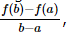a clearly positive value.
(v) Not always true, for example, the function might be strictly increasing guaranteeing the derivative to be always positive.
Thus, the true statements are (i), (ii) and (iv).

QUESTION: 43
If the sum of the first 2n terms of the A.P. 2, 5, 8,... is equalto the sum of the first n terms of the A.P. 57, 59, 61,.... then n equals
Solution:
QUESTION: 44

If f (x) = {2x - 3, x ≤ 2} {x, x < 2} then f (1) is equal to

Solution:
QUESTION: 45

If a, b, c, d, e, f are in A.P., then e - c is equal to

Solution:

Given a, b, c, d, e, f are in A.P.

Let p be the common difference.
b = a+p
c = a+2p
d = a+3p
e = a+4p
f = a+5p
d-c = a+3p – a-2p = p
e-c = a+4p-(a+2p) = 2p
= 2(d-c)

QUESTION: 46
The domain of definition of the function y (x) given by the equation 2x + 2y = 2 is
Solution:
QUESTION: 47

A line passing through point A(-5,-4) meet other three lines x + 3y + 2 = 0, 2x + y + 4 = 0 and x - y - 5 = 0 at B, C and D respectively. If ( 15 AB )2 + ( 10 AC )2 = ( 6 AD )2, then the equation of line is

Solution:

Equation of any line through A(-5, -4) is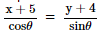= r(say)
then the coordinates of any point on this line at a distance r from A are
(r cosθ - 5, r sinθ - 4)
If AB = r1, AC = r2, AD = r3
then (r1 cosθ - 5, r1 sinθ - 4) lies on x + 3y + 2 = 0
⇒ r1 cosθ - 5 + 3 (r1 sinθ - 4) + 2 = 0
⇒ r1 (cosθ + 3 sinθ) = 15
⇒ r1 =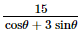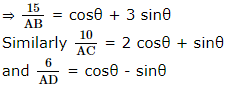Therefore according to the given condition
(cosθ + 3 sinθ)2 + (2 cosθ + sinθ)2
= (cosθ - sinθ)2
⇒ 4 cos2θ + 9 sin2θ + 12 sinθ cosθ = 0
⇒ (2 cosθ + 3 sinθ)2 = 0
⇒ 2 + 3 tanθ = 0
⇒ θ = -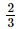Hence the required equation of the line is
y + 4 = -(x + 5)
or 2x + 3y + 22 = 0

QUESTION: 48
The ortho centre of the triangle formed by the lines x = 3, y = 4 and 3x + 4y = 6 is
Solution:
QUESTION: 49

If α1 , α2 … αn be the roots of the equation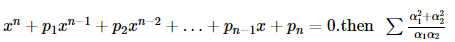is equal to_____

Solution: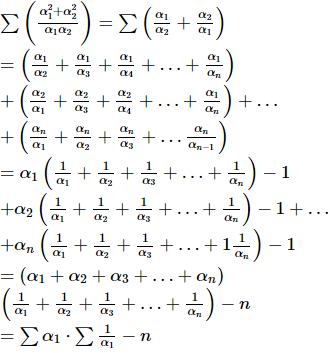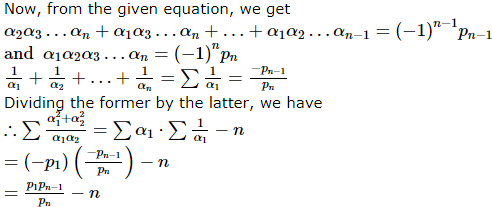QUESTION: 50

If a is any real number, the number roots of cot x − tan x = a in the first quadrant is

Solution:
QUESTION: 51

Evaluate the differentiation of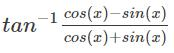Solution:

Explanation: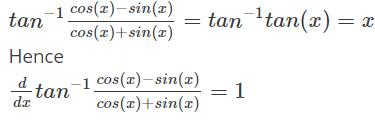QUESTION: 52

If the normals at (xi, yi), i = 1,2,3,4 on the rectangular hyperbola xy = c2 meet the point α , β then

Solution:
QUESTION: 53

Let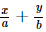= 1 and a x + b y = 1 be two variable lines and a, b be parameters such that a2 + b2 = ab then the locus of point of intersection of the lines is

Solution:QUESTION: 54

The determinant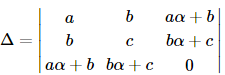is equal to zero if

Solution:
QUESTION: 55

The locus of the point of intersection of the lines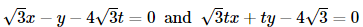(t is a parameter) is a hyperbola with eccentricity

Solution:
QUESTION: 56

{x} and [x] represent fractional and integral part of x, then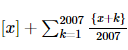is equal to

Solution:
QUESTION: 57

If 0 < x < 1, then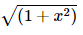[{x cos (cot-1 x) + sin (cot-1 x)}2 - 1]1/2 =

Solution:
QUESTION: 58

If p, q, r are positive integers and ω be an imaginary cube roots of unity and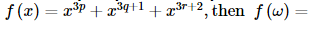Solution:
QUESTION: 59

The sum of the infinite terms of the series tan−1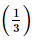+ tan−1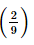+ tan−1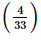+ … is equal to

Solution:
QUESTION: 60

If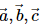are non-coplanar non-zero vectors and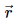is any vector in space then,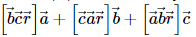is equal to

Solution:
QUESTION: 61

(ο) is a binary function such that aοb = a-b+1 where a,b∈z then the given binary function is

Solution:
QUESTION: 62

If a ∗ b = a+b-2 and if x ∗ 3 = 7 then what is the value of x-1?

Solution:
QUESTION: 63

The system of confocal conics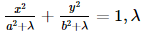being an arbitary constant

Solution:
QUESTION: 64

If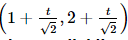be any point on a line then the range of values of t for which the point P lies between the parallel lines x + 2y = 1 and 2x + 4y = 15 is:

Solution:
QUESTION: 65

If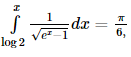, then the value of x is

Solution:
*Multiple options can be correct
QUESTION: 66

Which of the following expression are meaningful ?

Solution:
*Multiple options can be correct
QUESTION: 67

The polynomial expression (x3 + ax2 + bx + c)     is divisible by (x+ 1)2, then

Solution:
*Multiple options can be correct
QUESTION: 68

If y = f(x)  be the equation of a parabola (the axis of the parabola being parallel to the y-axis) which is touched by the line y = x at x = 1, then

Solution:
*Multiple options can be correct
QUESTION: 69

If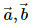are any two vectors then the vector bisecting the angle between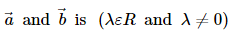Solution:
*Multiple options can be correct
QUESTION: 70

If 8 sinθ + 7 cosθ = 8 , then the value of 7 sin θ − 8 cosθ is equal to

Solution:
*Multiple options can be correct
QUESTION: 71

Let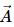be vector parallel to line of intersection of planes P1 and P2. Plane P1 is parallel tp the vectors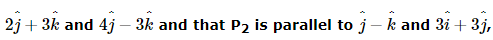then the angle between vectorand a given vector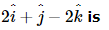Solution:
*Multiple options can be correct
QUESTION: 72

In Δ ABC, if tan A and tan B are the roots of the equation ab(x2 + 1) = c2x where a, b, c are the sides of the triangle, then

Solution:
*Multiple options can be correct
QUESTION: 73

If 3 unequal numbers a, b, c are in H.P. and their squares are in A.P., then a : b : c is equal to

Solution:
*Multiple options can be correct
QUESTION: 74

The function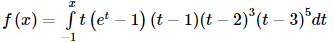has a local minimum at x

Solution:
*Multiple options can be correct
QUESTION: 75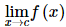does not exist when
Where [x] denotes step up function and {x} fractional part function

Solution:Use Code STAYHOME200 and get INR 200 additional OFF Use Coupon Code

Track your progress, build streaks, highlight & save important lessons and more!

Similar ContentRelated tests